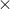In: Chemistry

# Determine the mass of oxygen in a 3.6 g sample of Al2(SO4)3. m(O) =

Determine the mass of oxygen in a 3.6 g sample of Al2(SO4)3.

m(O) =

## Solutions

##### Expert Solution

for the calculation of mass of oxygen in Al2(SO4)3, first calculate the no of moles of Al2(SO4)3 from 3.6g .

No of mole of Al2(SO4)3= given mass / molecular mass

=3.6 /342.15

=0.01 mol

Al2(SO4)3 has 12 Oxygen atoms,then the no of moles of oxygen = 120.01

=0.12 mol

mass of 0.12 mol Oxygen =0.1216     ( 16 is atomic mass of oxygen)

=1.92 g

## Related Solutions

##### How many moles of Al2(SO4)3 are there in 10.26 g of Al2(SO4)3?
How many moles of Al2(SO4)3 are there in 10.26 g of Al2(SO4)3?
##### (a) The reaction of Al2(SO4)3 solution with NaOH forms a precipitate according to the equation: Al2(SO4)3...
(a) The reaction of Al2(SO4)3 solution with NaOH forms a precipitate according to the equation: Al2(SO4)3 (aq) + 6NAOH (aq) >>> 3Na2SO4 + 2Al(OH)3. (i) Which species is the precipitate? (b) The addition of more NaOH causes the precipitate in the initial reaction to redissolve: Al(OH)3 + NaOH >>> NaAl(OH)4 (aq) (ii) Write the formula of the complex ion. (iii) Write the net ionic equation for this reaction. (c) Finally, addition of an acid HCl to the solution in (b)...
##### What is the TOTAL number of atoms in 10.26 g of Al2(SO4)3?
What is the TOTAL number of atoms in 10.26 g of Al2(SO4)3?
##### 9) for the reaction [ 2Al + 3H2SO4 → Al2(SO4)3 + 3 H2SO4] 6.0 g of...
9) for the reaction [ 2Al + 3H2SO4 → Al2(SO4)3 + 3 H2SO4] 6.0 g of Al (MW=26.98 g/mol) are added to 12.0 mL of 0.20 M of H2SO4 A) what is the limiting reagent in the above reaction? b) how many miles of H2SO4 will be formed? c) how many grams of Al2(SO4)3 (MW=342.15 g/mol) are produced in the reaction?
##### a) How many moles of Al2(SO4)3 are required to make 45 mL of a 0.080 M...
a) How many moles of Al2(SO4)3 are required to make 45 mL of a 0.080 M Al2(SO4)3 solution? (Hint, molarity is moles per liter. You know the molarity. You are given the number of mL's, which can be converted to liters. Just set it up so the units cancel and you get "moles".) _____________ moles Al2(SO4)3 b) What is the molar mass of Al2(SO4)3, to the nearest gram? _____________ [grams/mole] c) What mass of Al2(SO4)3 is required to make 45...
##### A 0.3999g sample of reagent grade Al2(SO4)3.xH2O was dissolved and analyzed for its aluminum content gravimetrically...
A 0.3999g sample of reagent grade Al2(SO4)3.xH2O was dissolved and analyzed for its aluminum content gravimetrically by precipitation as the aluminum oxinate complex, AL(C9H6NO)3, the precipitate weighing 0.4185g. Calculate the number of molecules of water of crystallization.
##### In the following reaction2Al(s) + 3H2SO4(aq) -------Al2(SO4)3(aq) + 3H2(g) When 13.5 gram of Al is reacted...
In the following reaction2Al(s) + 3H2SO4(aq) -------Al2(SO4)3(aq) + 3H2(g) When 13.5 gram of Al is reacted with 3.00 M solution of H2SO4, whatvolume of solution is required to react with all of the Al?
##### After dissolving 1.430g of a mixture of Na2SO4 and Al2(SO4)3 in water, you add an excess...
After dissolving 1.430g of a mixture of Na2SO4 and Al2(SO4)3 in water, you add an excess of BaCl2 solution. You filter off the preciptitate which forms, dry it, and find that it weighs 2.54g. What is the percent by mass of Al2(SO4)3 in the original mixture? The first person who answered this was wrong. The answer is supposed to be 33% but can someone show me how?
##### Consider the following unbalanced equation for the reaction of aluminum with sulfuric acid. Al(s)+H2SO4(aq)→Al2(SO4)3(aq)+H2(g). How many...
Consider the following unbalanced equation for the reaction of aluminum with sulfuric acid. Al(s)+H2SO4(aq)→Al2(SO4)3(aq)+H2(g). How many moles of H2 are formed by the complete reaction of 0.384 mol of Al? Express your answer using three significant figures. Chemical Equation -> 2C4H10(g)+13O2(g)→10H2O(g)+8CO2(g) Calculate the mass of water produced when 2.66 g of butane reacts with excess oxygen. Express your answer to three significant figures and include the appropriate units. Calculate the mass of butane needed to produce 62.7 g of carbon...
##### A gaseous compound is 30.4% nitrogen and 69.6% oxygen by mass. A 5.25-g sample of the...
A gaseous compound is 30.4% nitrogen and 69.6% oxygen by mass. A 5.25-g sample of the gas occupies a volume of 1.00 L and exerts a pressure of 1.26 atm at -4.0°C. What are the empirical and molecular formulas, respectively? Show how you determined the molar mass.